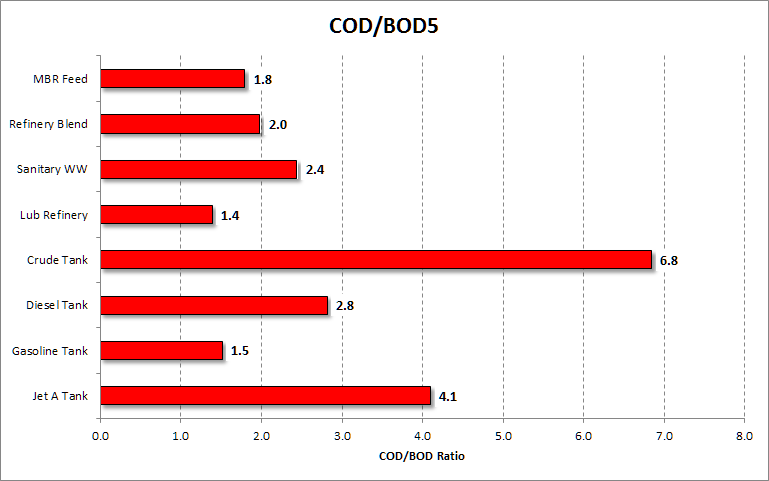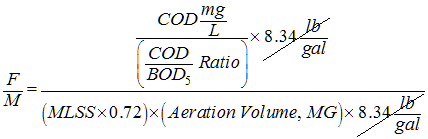top of page

# Food-to-Mass (F:M) Ratio

Updated: Apr 4, 2021

### Introduction

Before going into a detailed discussion of the F:M (food-to-mass or food-to-microorganism) ratio I want to let you know about changing views toward this process control parameter. Here are two opposing examples, the first from 1988 and the second from 2011.

==

This ratio, called the food-to-microorganism or food/mass (F/M) ratio, can be measured and is an important factor in design and operation of aeration basins, such as activated sludge basins.

Source: The Nalco Water Handbook (2nd ed.). (1998).

==

The parameter of fundamental importance in the design and control of the activated sludge system is the sludge age, which governs the mass of sludge to be wasted daily from the system. The sludge age can and should replace completely the Food to Microorganism ratio (F/M, kg BOD or COD load per day per kg MLSS or MLVSS in reactor) or equivalently the Load Factor (LF) as a reference and control parameter, in particular if nitrification is required.

Source: Henze, M., van Loosdrecht, M. C. M., Ekama, G. A., & Brdjanovic, D. (2011). Biological Wastewater Treatment: Principles, Modelling and Design.

==

### My View

When the data is available, and it usually is, I always take a look at the F:M ratio. Perhaps I'm "old school" in that I continue to be interested in this parameter. But I'm always interested in observing trends and extremes in any data set and doing comparisons to observe relationships between variables. I want to look at, and analyze, all the available data before I reject or discard information if it doesn't seem relevant or useful. For example, look at the graph below, which shows the F:M ratio at a refinery with a complete mix activated sludge system. The optimal range for the F:M ratio in a complete mix system is 0.2 to 0.6 lb BOD/lb MLVSS (kg BOD/kg MLVSS).

The first 69 days of data are interesting because of the amount of variability shown in the F:M ratio. The graph indicates the activated sludge process is not in control during this period. From day 70 on the process seems to stabilize though periodically bumping up against the recommended limit of 0.6.The graph below is the same graph as above but effluent COD (chemical oxygen demand) data has been added. Does a high F:M ratio lead to a higher effluent COD concentration? Sometimes but not always, so I don't think we can use the F:M ratio as a predictor of effluent COD.The graph below, generated using Minitab (statistical analysis software), takes a more formal look at the relationship between the F:M ratio and effluent COD concentration using regression analysis. The R-Squared value, at just 5.8% , indicates there is little correlation between the two variables. In other words, with this particular set of data you won't be able to use the F:M ratio as a means to predict the effluent chemical oxygen demand concentration.You will have to decide for yourself how you want to use the Food-to-Microorganism ratio and the value you place in the information it provides. To that end what follows is a detailed exploration of this activated sludge process control parameter.

### F:M (Food-to-Mass) Ratio

For the activated sludge process to operate properly there must be a balance between the food entering the bioreactor and the microorganisms in the bioreactor. A high F:M ratio means there is a greater quantity of food (measured as BOD, COD, or TOC) relative to the quantity of microorganisms available to consume that food. When the F:M ratio is high, the bacteria are active and multiply rapidly but they are also more dispersed in suspended growth bioreactors. Because of that dispersion, a high F:M ratio creates an environment where the bacteria will not form a good, large, dense floc. For that reason, a high F:M ratio will often result in a poor settling sludge in the secondary clarifier and a turbid effluent.

A low F:M ratio means there are many microorganisms but there is a limited amount of food. Only when the food supply is limited do bacteria begin to develop a thicker slime layer, lose their motility, and begin to clump together to form a dense floc that will settle well in the clarifier. The graphic below shows how the bacterial population relates to the food supply. As you can see, a very high F:M ratio, or a very low F:M ratio will result in dispersed floc that will not settle well in the secondary clarifier.

Calculating the F:M ratio is easy in terms of the formula but obtaining accurate inputs significantly increases the difficulty of determining the “true” F:M ratio. I’ll discuss this in detail a bit later. In order to calculate the F:M ratio two quantities are required: 1) the pounds (kilograms) of organic material entering the bioreactor and 2) the pounds (kilograms) of microorganisms in the bioreactor.

The standard equation for calculating the food-to-mass (microorganism) ratio is shown in the equation below. Note that in this equation the organic load, the food, is based on the five-day biochemical oxygen demand (BOD5) concentration and the microorganism concentration is based on the mixed liquor volatile suspended solids (MLVSS) concentration. This is the most common form of the F:M ratio equation where "MG" is million gallons.

Formula for the F:M RatioThough the F:M equation is uncomplicated, it does have two variables, highlighted in red above, that can pose a potential problem. If you are a municipal wastewater treatment plant you will very likely have all your organic concentration data measured as BOD5 which you can plug directly into the equation. But if you are an industrial wastewater plant you will typically have your organic concentrations measured as chemical oxygen demand (COD) or perhaps, even, as total organic carbon (TOC). You cannot simply replace the BOD concentration with the COD or TOC concentration in the formula above in order to determine if you are operating in an acceptable range for your type of bioreactor, as shown in the table below.

### COD/BOD5 Ratio

In order to use COD or TOC values in place of BOD5 you really need to know the ratio of your COD to BOD (or TOC to BOD). The table below shows that the COD/BOD ratio is commonly referenced as being 2.1 but this is an approximate value that will fit better with municipal wastewater. As the industrial contribution increases to a municipal wastewater plant the COD/BOD ratio is likely to increase. But by how much? For strict industrial wastewater plants the COD/BOD ratio can be highly variable, increasing significantly beyond the oft-used 2.1 ratio value. This is important because "wastewater with a high COD to BOD ratio indicates that a substantial part of the organic matter will be difficult to degrade biologically" (Henze, et al., 2008).

The table and bar graph below will give you an idea of just how variable the COD/BOD ratio can be. In the table, the COD/BOD ratio ranges from 1.4 to 6.8. The bar graph uses the COD/BOD ratio data from the table. The takeaway from this is that the COD/BOD ratio is not only variable, it is also plant and waste stream specific. Often, an industrial plant won't have both BOD and COD data so the true ratio cannot easily (immediately) be determined. In that case, without BOD data, you'll have to apply the factor you think might best quantify your COD/BOD ratio. Sending samples out for COD and BOD testing periodically will help you dial in your plant's ratio. With confidence in the value of your COD/BOD ratio you can convert COD concentrations to BOD equivalents for use in the F:M equation. This is easier said than done!In the graph below you can see a curve fitted to influent COD/BOD data from a refinery. The data was collected over a period of three years and includes 1,306 data points. This particular refinery does an extraordinary job of collecting wastewater samples and analyzing for numerous parameters. The graph shows that 90% of the COD/BOD ratios fall between 2.51 and 6.36. The mean COD/BOD ratio for this data set is 3.96. Without this data, using a COD/BOD value of 2.1 in its place would result in a rather complete mis-characterization of the true BOD concentration for this industrial wastewater system.### True Concentration of Viable Biomass

The next issue with the F:M ratio arises in determining the "true" quantity of healthy, functioning microorganisms that are available to oxidize organics. The F:M equation requires the use of the mixed liquor suspended solids (MLVSS) concentration to estimate the population of viable biomass. Using the MLVSS, rather than the MLSS, is intended to provide a better estimate of the microorganism concentration because inert material is removed from the MLVSS value. But the MLVSS still overestimates the microorganism population because the value includes both living and dead biomass in addition to inert organic material. One solution is to use adenosine triphosphate testing to accurately determine the "viable" microorganism concentration. I discuss this in detail on another page about ATP testing.

So far I have assumed that the MLVSS concentration is available. Here again, a distinction between municipal and industrial wastewater plants needs to be made. If you are a municipal wastewater plant, it is highly likely you have a wealth of MLSS and MLVSS data. If you are an industrial wastewater system, it is possible that you do not have MLVSS data. If you don't have MLVSS data you can use the graph below to estimate this concentration. I recommend you assume the MLVSS to comprise 72% of the MLSS concentration.The COD-based F:M ratio equation now looks like this:Tags:

bottom of page